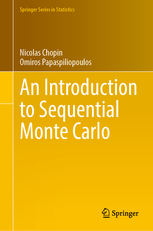# BookAn introduction to Sequential Monte Carlo

Nicolas Chopin and Omiros Papaspiliopoulos

Available here. See software for the accompanying Python library, particles.

Chapters:

1. Introduction
2. Introduction to state-space models
3. Beyond state-space models
4. Introduction to Markov processes
5. Feynman-Kac models: definition, properties and recursions
6. Finite state-spaces and hidden Markov models
7. Linear-Gaussian state-space models
8. Importance sampling
9. Importance resampling
10. Particle filtering
11. Convergence and stability of particle filters
12. Particle smoothing
13. Sequential quasi-Monte Carlo
14. Maximum likelihood estimation of state-space models
15. Markov chain Monte Carlo
16. Bayesian estimation of state-space models and particle MCMC
17. SMC samplers
18. SMC^2, sequential inference in state-space models
19. Advanced topics and open problems

Typos: see here.